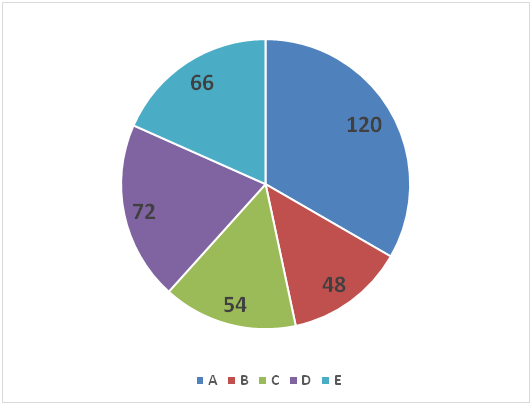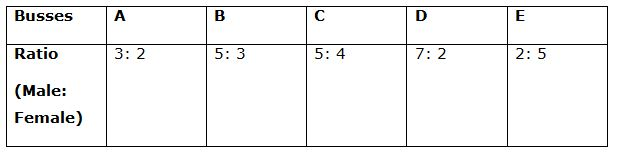# IBPS RRB Clerk Mains Quantitative Aptitude Questions 2019 (Day-08)

Dear Aspirants, Our IBPS Guide team is providing new series of Reasoning Questions for IBPS RRB Clerk Mains 2019 so the aspirants can practice it on a daily basis. These questions are framed by our skilled experts after understanding your needs thoroughly. Aspirants can practice these new series questions daily to familiarize with the exact exam pattern and make your preparation effective.

Check here for IBPS RRB PO Mains Mock Test 2019

Check here for IBPS RRB Clerk Mains Mock Test 2019

Check here for IBPS PO Prelims Mock Test 2019

### Click Here to Subscribe Crack High Level Puzzles & Seating Arrangement Questions PDF 2019 Plan

[WpProQuiz 7197]

Directions (1 – 5): Study the following information carefully and answer the questions given below:

There are five different busses A, B, C, D and E that are travelling between two cities P and Q. Pie chart given below shows the distribution (in degree) of number of total passengers travelling in those two busses respectively.

Note: Ratio per male passenger fare to the per female passenger fare is same for all the busses which is equal to 5: 3.

Sum of pie chart = 1260 passengers1) If total amount of fare collected from the passengers in bus A is Rs.9300 and number of male passengers in bus A and bus D is 180 and 52 more than the female passengers respectively. What is the total amount of fare collected from the passengers in bus D if ratio of per male passenger fare in bus A to that in bus D is 5: 4?

a) Rs.3620

b) Rs.2750

c) Rs.4240

d) Rs.3850

e) None of these

2) If the ratio of number of male passengers to female passengers travelling in bus D is 5: 4 and the ratio of male passengers to female passengers travelling in bus E is6: 5, then what is the ratio of sum of male passengers travelling in bus D and number of female passengers  travelling in bus E together to the sum of number of female passengers travelling in bus D and number of male passengers travelling in bus E together?

a) 35: 34

b) 5: 4

c) 15: 14

d) 25: 24

e) None of these

3) If number of male passengers travelling in another bus F is 25% more than the total number of passengers travelling in bus B. Ratio of male passengers to female passengers travelling in bus F is 4: 3, then what is the difference between total number of passengers travelling in bus F and that travelling in bus A?

a) 48

b) 36

c) 42

d) 32

e) None of these

4) If total number of passengers travelling in all the five busses when all the busses are travelling between cities X and Y together is 1080 and out of which 15% are travelling in bus C and out of remaining passengers, (2/9)th are travelling in bus E, then what is the difference between total number of passengers travelling in buses C and E together when travelling between cities P and Q and total number of passengers travelling in buses C and E together when travelling between cities X and Y?

a) 66

b) 84

c) 54

d) 24

e) None of these

5) Table given below shows the ratio of male passengers to female passengers travelling in the five different busses, then what is the difference between the total number of male passengers travelling in busses A and B together and total number of female passengers travelling in busses C, D and E together?a) 23

b) 31

c) 26

d) 18

e) None of these

Direction (6 – 10): What value should come in the place of question mark in the given series?

6) 2209, 1849, 1681, 1369, 961, 841, ?

a) 529

b) 729

c) 625

d) 441

e) 576

7) 19, 23, 32, 48, 73, 109, ?

a) 162

b) 158

c) 170

d) 148

e) 126

8) 23, 34.5, 69, 172.5, 517.5, 1811.25, ?

a) 7250

b) 7234

c) 7245

d) 7256

e) 7124

9) 599, 722, 968, 1337, 1829, 2444, ?

a) 3182

b) 2896

c) 3459

d) 2997

e) 4256

10) 11, 43, 170, 677, 2704, 10811, ?

a) 43838

b) 45238

c) 43278

d) 43238

e) 41278

Directions (1-5) :

Letper male passenger fare and per female passenger fare for all the busses is 5x and 3x respectively.

Total passengers in bus A = 1260 * (120/360) = 420

Total passengers in bus D = 1260 * (72/360) = 252

Let male and female passengers in bus A is ‘a’ and ‘a – 180’ respectively.

a + (a – 180) = 420

a = 300

Male and female passengers in bus A is 300 and 120 respectively.

According to the question:

(300 * 5x) + (120 * 3x) = 9300

1860x = 9300

x = 5

Let male and female passengers in bus D is ‘d’ and ‘d – 180’ respectively.

d + (d – 52) = 252

d = 152

Male and female passengers in bus D is 152 and 100 respectively.

Per male passenger fare for bus D = 5x * (4/5) = 4x = Rs.20

Per female passenger fare for bus D = 20 * (3/5) = Rs.12

Total amount of fare collected from the passengers in bus D = (152 * 20) + (100 * 12) = Rs.4240

Total passengers in bus D = 1260 * (72/360) = 252

Male passengers in bus D = 252 * (5/9) = 140

Female passengers in bus D = 252 * (4/9) = 112

Total passengers in bus E = 1260 * (66/360) = 231

Male passengers in bus E = 231 * (6/11) = 126

Female passengers in bus E = 231 * (5/11) = 105

Sum of male passengers travelling in bus D and number of female passengers travelling in bus E together = 140 + 105 = 245

Sum of female passengers travelling in bus D and number of male passengers travelling in bus E together = 112 + 126 = 238

Required ratio = 245: 238 = 35: 34

Total passengers in bus A = 1260 * (120/360) = 420

Total passengers in bus B = 1260 * (48/360) = 168

Total male passengers in bus F = 125% of 168 = 210

Total female passengers in bus F = 210 * (4/5) = 168

Total passengers in bus F = 210 + 168 = 378

Required difference = 420 – 378 = 42

Total number of passengers in buses C and E together when travelling between cities P and Q = 1260 * [(54 + 66)/360] = 420

Total number of passengers in bus C when travelling between cities X and Y = 15% of 1080 = 162

Total number of passengers in bus E when travelling between cities X and Y = (2/9) of (1080 – 162) = 204

Total number of passengers in buses C and E together when travelling between cities X and Y = 162 + 204 = 366

Required difference = 420 – 366 = 54

Male passengers in buss A = 1260 * (120/360) * (3/5) = 252

Male passengers in buss B = 1260 * (48/360) * (5/8) = 105

Female passengers in buss C = 1260 * (54/360) * (5/9) = 105

Female passengers in buss D = 1260 * (72/360) * (2/9) = 56

Female passengers in buss E = 1260 * (66/360) * (5/7) = 165

Total number of male passengers travelling in busses A and B together = 252 + 105 = 357

Total number of female passengers travelling in busses C, D and E together = 105 + 56 + 165 = 326

Required difference = 357 – 326 = 31

Directions (6-10) :

Square of prime numbers

2209 = 47 * 47

1849 = 43 * 43

1681 = 41 * 41

1369 = 37 * 37

961 = 31 * 31

841 = 29 * 29

529 = 23 * 23

19 + 22 = 23

23 + 32 = 32

32 + 42 = 48

48 + 52 = 73

73 + 62 = 109

109 + 72 = 158

23 * 1.5 = 34.5

34.5 * 2 = 69

69 * 2.5 = 172.5

172.5 * 3 = 517.5

517.5 * 3.5 = 1811.25

1811.25 * 4 = 7245

599 + (1 * 123) = 722

722 + (2 * 123) = 968

968 + (3 * 123) = 1337

1337 + (4 * 123) = 1829

1829 + (5 * 123) = 2444

2444 + (6 * 123) = 3182

11 * 4 – 1 = 43

43 * 4 – 2 = 170

170 * 4 – 3 = 677

677 * 4 – 4 = 2704

2704 * 4 – 5 = 10811

10811 * 4 – 6 = 43238